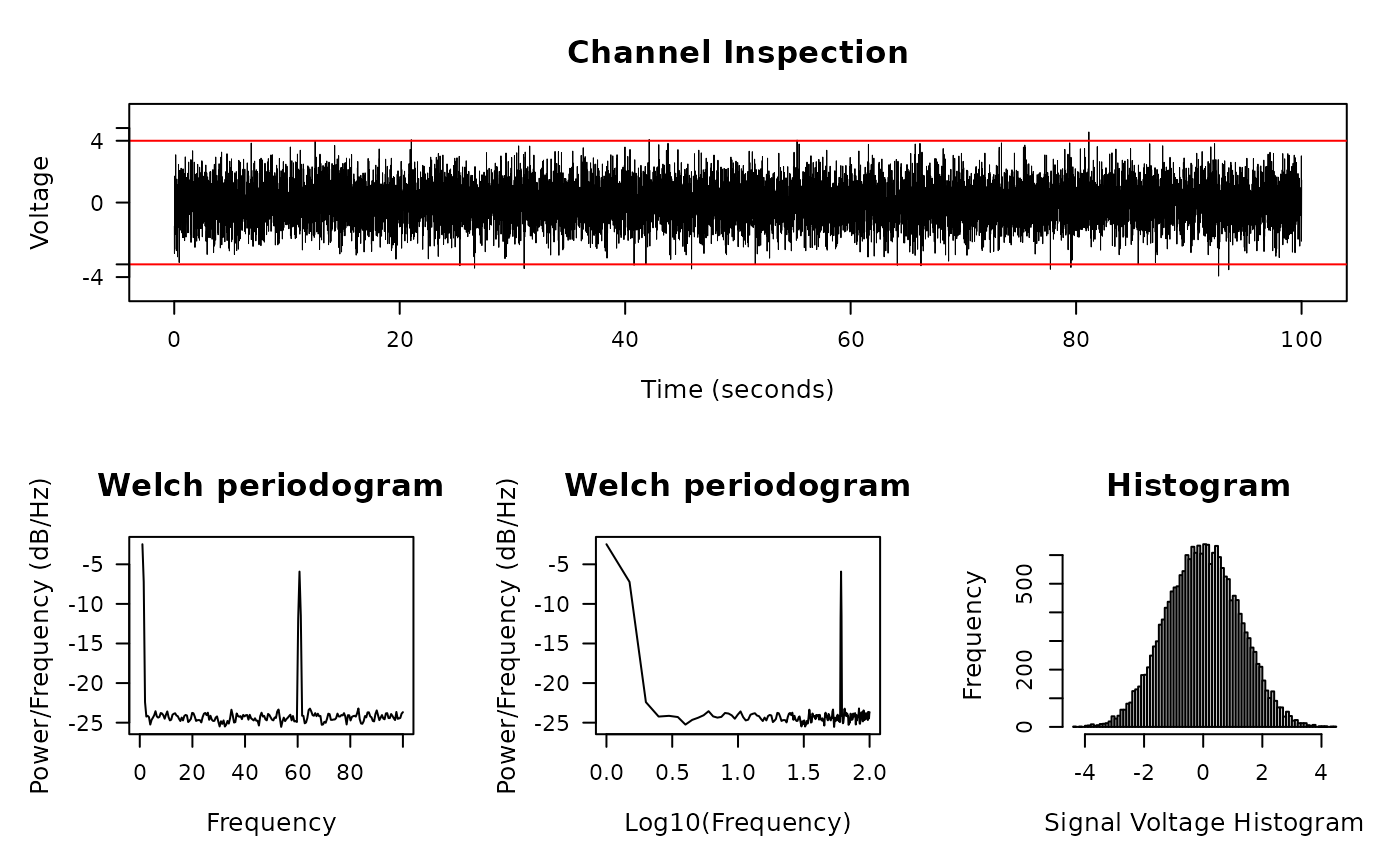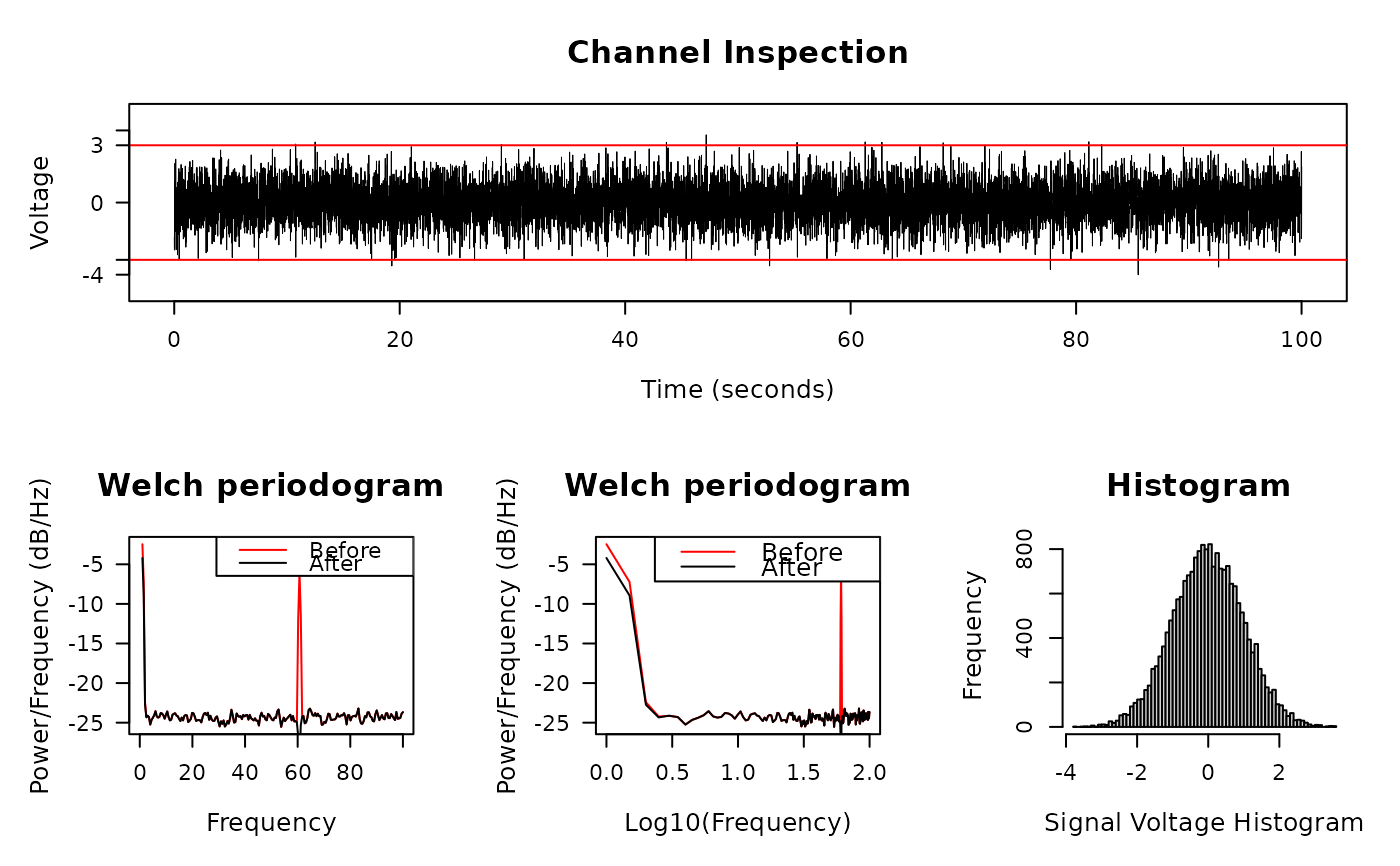Plot and Inspect Signals in Trace, Periodogram, and Histogram

diagnose_signal(
s1,
s2 = NULL,
sc = NULL,
srate,
name = "",
try_compress = TRUE,
max_freq = 300,
window = ceiling(srate * 2),
noverlap = window/2,
std = 3,
cex = 1.5,
lwd = 0.5,
flim = NULL,
nclass = 100,
main = "Channel Inspection",
col = c("black", "red"),
which = NULL,
start_time = 0,
boundary = NULL,
mar = c(5.2, 5.1, 4.1, 2.1),
...
)

## Arguments

s1 Signal for inspection Signal to compare, default NULL compressed signal to speedup the trace plot, if not provided, then either the original s1 is used, or a compressed version will be used. See parameter try_compress. Sample rate of s1, note that s2 and s1 must have the same sample rate Analysis name, for e.g. "CAR", "Notch", etc. If length of s1 is too large, it might take long to draw trace plot, my solution is to down-sample s1 first (like what Matlab does), and then plot the compressed signal. Some information will be lost during this process, however, the trade-off is the speed. try_compress=FALSE indicates that you don't want to compress signals under any situation (this might be slow). Max frequency to plot, should be no larger than half of the sampling rate. Window length to draw the Periodogram Number of data points that each adjacent windows overlap Error bar (red line) be drawn at standard deviations, by default is 3, meaning the error bars represent 3 standard deviations. passed to plot.default log10 of frequency range to plot Number of classes for histogram Plot title Color for two signals, length of 2. Which sub-plot to plot When does signal starts Boundary for signal plot, default is 1 standard deviation

## Examples

library(stats)
time <- seq(0, 100, by = 1/200)
s2 <- sin(2 * pi * 60 * time) + rnorm(length(time))
diagnose_signal(s2, srate = 200)#> $ylim #>  4.431596 #> #>$boundary
#>  3.674513
#>

# Apply notch filter
s1 = notch_filter(s2, 200, 58,62)
diagnose_signal(s1, s2, srate = 200)#> $ylim #>  3.755492 #> #>$boundary
#>  2.98199
#>